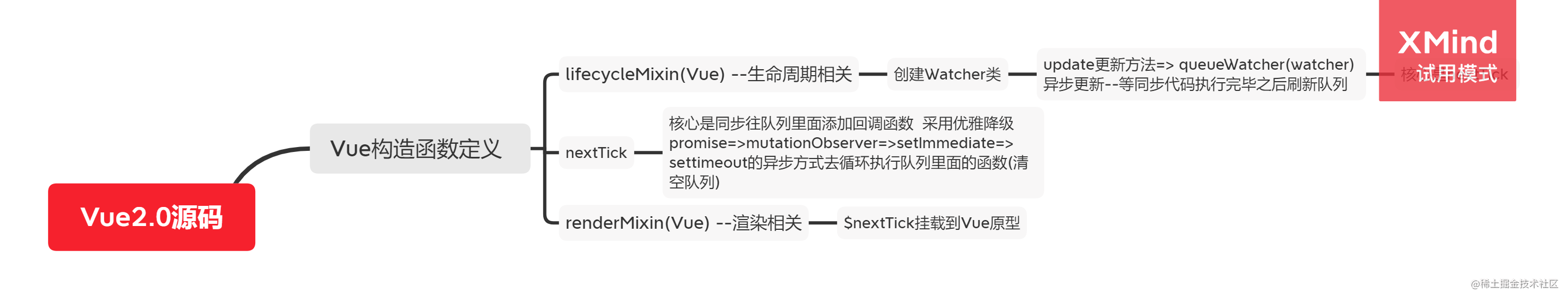### 正文

``````<script>
// Vue实例化
let vm = new Vue({
el: "#app",
data() {
return {
a: 123,
};
},
// render(h) {
//   return h('div',{id:'a'},'hello')
// },
template: `<div id="a">hello {{a}}</div>`,
});

// 当我们每一次改变数据的时候  渲染watcher都会执行一次 这个是影响性能的
setTimeout(() => {
vm.a = 1;
vm.a = 2;
vm.a = 3;
}, 1000);
</script>
``````

#### 1.watcher 更新的改写

``````// src/observer/watcher.js

import { queueWatcher } from "./scheduler";
export default class Watcher {
update() {
// 每次watcher进行更新的时候  是否可以让他们先缓存起来  之后再一起调用
// 异步队列机制
queueWatcher(this);
}
run() {
// 真正的触发更新
this.get();
}
}
``````

#### 2.queueWatcher 实现队列机制

``````// src/observer/scheduler.js

import { nextTick } from "../util/next-tick";
let queue = [];
let has = {};
function flushSchedulerQueue() {
for (let index = 0; index < queue.length; index++) {
//   调用watcher的run方法 执行真正的更新操作
queue[index].run();
}
// 执行完之后清空队列
queue = [];
has = {};
}

// 实现异步队列机制
export function queueWatcher(watcher) {
const id = watcher.id;
//   watcher去重
if (has[id] === undefined) {
//  同步代码执行 把全部的watcher都放到队列里面去
queue.push(watcher);
has[id] = true;
// 进行异步调用
nextTick(flushSchedulerQueue);
}
}
}
``````

#### 3.nextTick 实现原理

``````// src/util/next-tick.js

let callbacks = [];
let pending = false;
function flushCallbacks() {
pending = false; //把标志还原为false
// 依次执行回调
for (let i = 0; i < callbacks.length; i++) {
callbacks[i]();
}
}
let timerFunc; //定义异步方法  采用优雅降级
if (typeof Promise !== "undefined") {
// 如果支持promise
const p = Promise.resolve();
timerFunc = () => {
p.then(flushCallbacks);
};
} else if (typeof MutationObserver !== "undefined") {
// MutationObserver 主要是监听dom变化 也是一个异步方法
let counter = 1;
const observer = new MutationObserver(flushCallbacks);
const textNode = document.createTextNode(String(counter));
observer.observe(textNode, {
characterData: true,
});
timerFunc = () => {
counter = (counter + 1) % 2;
textNode.data = String(counter);
};
} else if (typeof setImmediate !== "undefined") {
// 如果前面都不支持 判断setImmediate
timerFunc = () => {
setImmediate(flushCallbacks);
};
} else {
// 最后降级采用setTimeout
timerFunc = () => {
setTimeout(flushCallbacks, 0);
};
}

export function nextTick(cb) {
// 除了渲染watcher  还有用户自己手动调用的nextTick 一起被收集到数组
callbacks.push(cb);
if (!pending) {
// 如果多次调用nextTick  只会执行一次异步 等异步队列清空之后再把标志变为false
pending = true;
timerFunc();
}
}
``````

#### 4.\$nextTick 挂载原型

``````// src/render.js

import { nextTick } from "./util/next-tick";

export function renderMixin(Vue) {
// 挂载在原型的nextTick方法 可供用户手动调用
Vue.prototype.\$nextTick = nextTick;
}
``````

#### 4.异步更新的思维导图